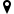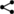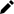Welcome guest
You're not logged in.
300 users online, thereof 1 logged in

## Example: Difference Operators of Some Functions

We have seen so far that the difference operator of falling factorial powers of the identity function $g(x)=x$ equals $\Delta x^{\underline n}=nx^{\underline {n-1}}$ for all integers $n\in\mathbb Z.$ There are many other special formulas of the difference calculus and they often, but not always, correspond to the respective statements for usual derivatives.

For instance, if $f(x)=a+bx,$ then
\begin{align} (a+bx)^{\underline n}&=(a+bx)(a+b(x-1))\cdots(a+b(x-n+1))\nonumber\\ \Delta(a+bx)^{\underline n}&=(a+b(x+1))(a+bx)\cdots(a+b(x-n+2))\nonumber\\ &\quad-(a+bx)(a+b(x-1))\cdots(a+b(x-n+1))\nonumber\\ &=bn[(a+b(x-1))\cdots(a+b(x-n+2))]\nonumber\\ &=bn(a+bx)^\underline{n-1}\nonumber\\ \end{align}

which is pretty similar to the corresponding formula for derivatives $\frac d{dx} (a+bx)^n=bn(a+bx)^{n-1}.$

The simple proofs of the other examples given in the table below are left to the reader.

Brief table of the difference operator Corresponding table of derivatives
$\Delta c=0$ $\frac d{dx} c=0$
$\Delta x^{\underline n}=nx^{\underline {n-1}}$ $\frac d{dx} x^n=nx^{n-1}$
$\Delta(a+bx)^{\underline n}=bn(a+bx)^\underline{n-1}$ $\frac d{dx} (a+bx)^n=bn(a+bx)^{n-1}$
$\Delta a^x=a^x(a-1)$ $\frac d{dx} a^x=a^x\log a$
$\Delta 2^x=2^x$ $\frac d{dx} e^x=e^x$
$\Delta \log_a(x)=\log_a\left(1+\frac 1x\right)$ $\frac d{dx} \log_a(x)=\frac 1{x\cdot\ln(a)}$
$\Delta \sin(ax)=2\sin\left(a/2\right)\cos\left(a(x+1/2)\right)$ $\frac d{dx} \sin(ax)=a\cos(ax)$
$\Delta \cos(ax)=-2\sin\left(a/2\right)\sin\left(a(x+1/2)\right)$ $\frac d{dx} \cos(x)=-a\sin(ax)$

The formulas for derivatives are given only for the convenience to easily compare the formulas between the difference calculus and “usual” calculus.

Please note that for the exponentiation, the basis $2$ has the same unique property for the difference operator as the Euler constant $e$ has for the derivative, namely the property that the respective operators difference and derivative do not change the respective functions $2^x$ and $e^x.$|||| created: 2020-04-03 15:37:27 | modified: 2020-05-16 16:17:44 | by: bookofproofs | references: , ,